Suppose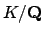is a quadratic field. Then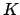is Galois, so for each prime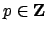we have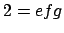. There are exactly three possibilties:
• Ramified: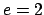,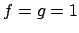: The prime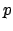ramifies in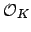, so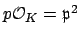. There are only finitely many such primes, since if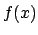is the minimal polynomial of a generator for, thenramifies if and only ifhas a multiple root modulo. However,has a multiple root moduloif and only ifdivides the discriminant of, which is nonzero becauseis irreducible over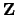. (This argument shows there are only finitely many ramified primes in any number field. In fact, we will later show that the ramified primes are exactly the ones that divide the discriminant.)
• Inert: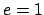,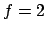,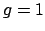: The primeis inert in, so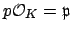is prime. This happens 50% of the time, which is suggested by quadratic reciprocity (but not proved this way), as we will see illustrated below for a particular example.
• Split: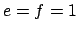,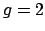: The primesplits in, in the sense that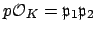with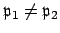. This happens the other 50% of the time.
Suppose, in particular, that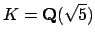, so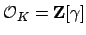, where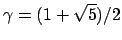. Then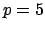is ramified, since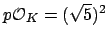. More generally, the order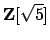has index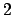in, so for any prime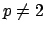we can determine the factorization ofinby finding the factorization of the polynomial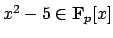. The polynomial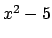splits as a product of two distinct factors in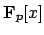if and only ifand. For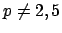this is the case if and only if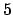is a square in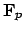, i.e., if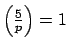, where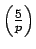is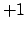ifis a square modand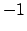ifis not. By quadratic reciprocity,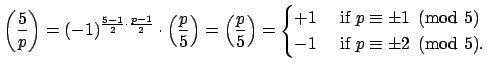Thus whethersplits or is inert inis determined by the residue class ofmodulo.